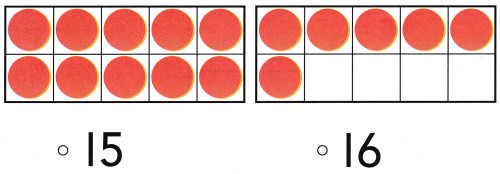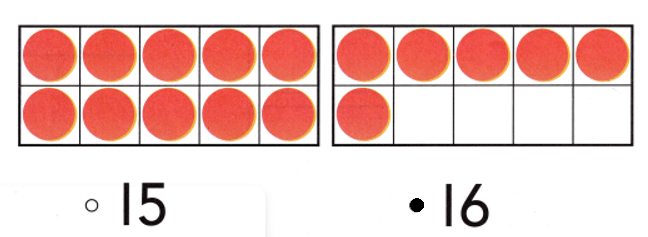# Texas Go Math Kindergarten Lesson 8.1 Answer Key Model and Count 16 and 17

Refer to our Texas Go Math Kindergarten Answer Key Pdf to score good marks in the exams. Test yourself by practicing the problems from Texas Go Math Kindergarten Lesson 8.1 Answer Key Model and Count 16 and 17.

## Texas Go Math Kindergarten Lesson 8.1 Answer Key Model and Count 16 and 17

Explore

DIRECTIONS: Use counters to show the number 16. Add more to show the number 17. Draw the counters.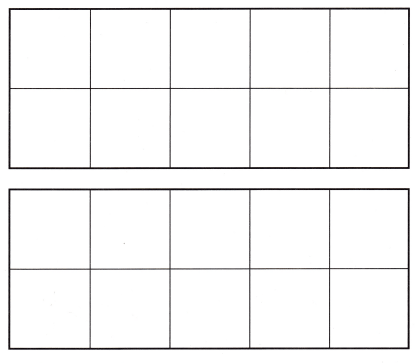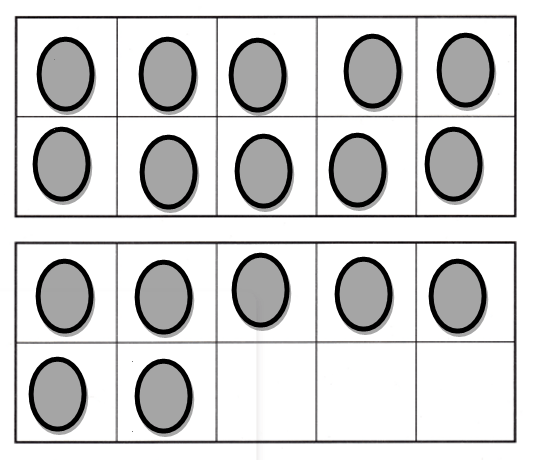Explanation:
Number of counters = 16.
One more than 16 = 16 + 1 = 17.

Share and Show

DIRECTIONS: 1. Circle 10 peaches. Tell how many more there are. 2. Place counters in the ten frames to show the number 16. Draw the counters. 3. Look at the counters you drew in the ten frames. How many ones are in the top ten frame? Write the number. How many ones are in the bottom ten frame? Write the number.

Question 1.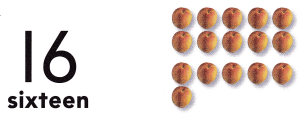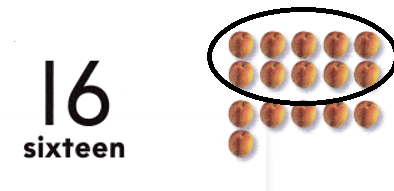Explanation:
Total number of peaches = 10 + 6 = 16 or Sixteen.

Question 2.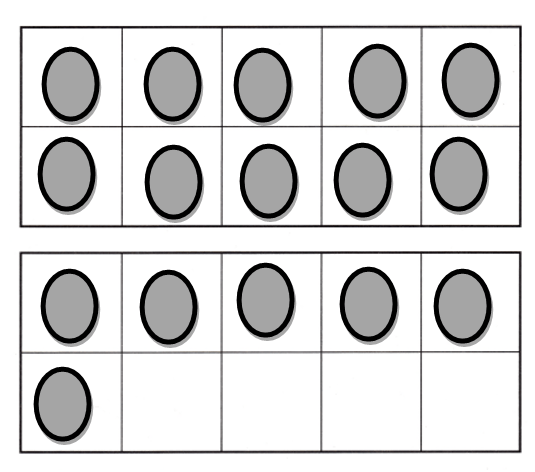Explanation:
Number of counters in the frame = 10 + 6
= 16 or Sixteen.

Question 3.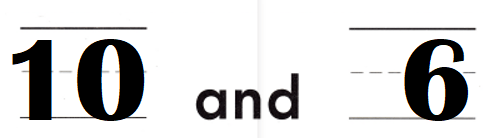Explanation:
Number of counters in the up of the frame = 10.
Number of counters in the down of the frame = 6.
Total number of counters  = Number of counters in the up of the frame + Number of counters in the down of the frame
= 10 + 6
= 16 or Sixteen.

DIRECTIONS: 4. Circle 10 peaches. Tell how many more there are. 5. Place and draw counters in the ten frames to show the number 17. 6. Look at the ten frames. Write the number of ones in the top ten frame. Write the number of ones in the bottom ten frame.

Question 4.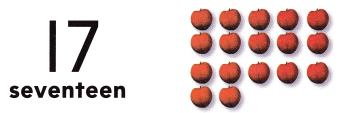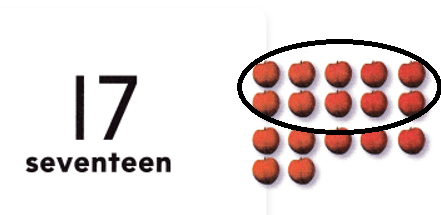Explanation:
Number of peaches = 10 + 7 = 17 or Seventeen.

Question 5.Explanation:
Number of counters = 10 + 7 = 17 or Seventeen.

Question 6.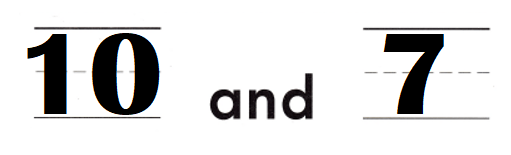Explanation:
Number of counters in the up of the frame = 10.
Number of counters in the down of the frame = 7.
Total number of counters  = Number of counters in the up of the frame + Number of counters in the down of the frame
= 10 + 7
= 17 or Seventeen.

HOME ACTIVITY • Draw two ten frames. Have your child use small objects to show the numbers 16 and 17.
Number of counters = 17 or Seventeen.Explanation:
My kid took yellow counters and placed them in the frames.
Number of counters = 16 or Sixteen.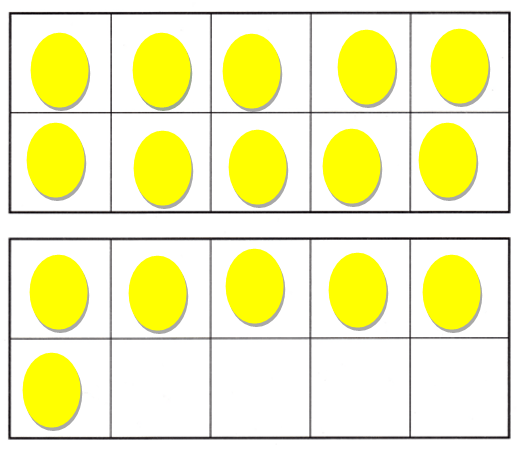Problem Solving
Question 7.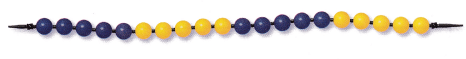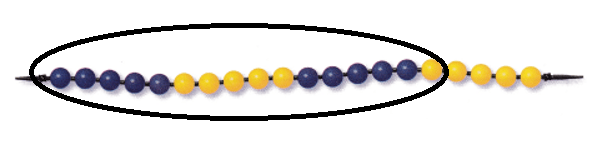Explanation:
Number of blue beads = 10.
Number of yellow beads = 6.
= 10 + 6
= 16 or Sixteen.

Question 8.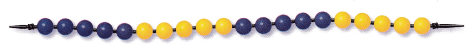Explanation:
Number of blue beads = 10.
Number of yellow beads = 7.
= 10 + 7
= 17 or Seventeen.

Question 9.Explanation:
Number of counters = 17 or Seventeen.

### Texas Go Math Kindergarten Lesson 8.1 Homework and Practice Answer Key

DIRECTIONS: 1. Draw counters to show 16. Write the number. 2. Draw counters to show 17. Write the number.

Question 1.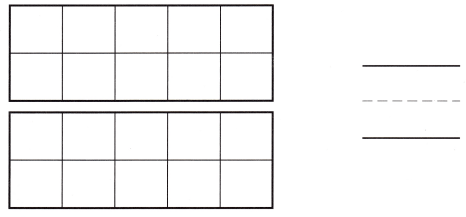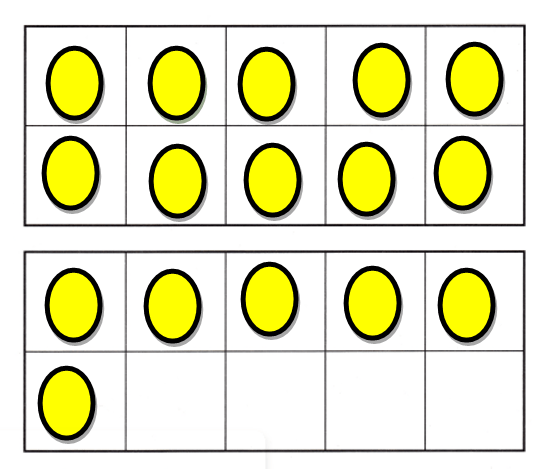Explanation:
Number of counters = 16 or Sixteen.

Question 2.Explanation:
Number of counters = 17 or Seventeen.

DIRECTIONS: Choose the correct answer. 3-5. What number does the model show?

Lesson Check
Question 3.Explanation:
Number of counters = 16 or Sixteen.

Question 4.Explanation:
Number of counters = 17 or Seventeen.

Question 5.